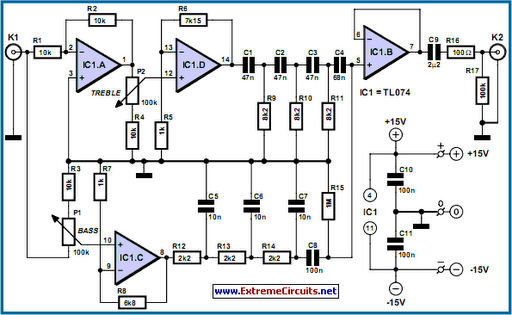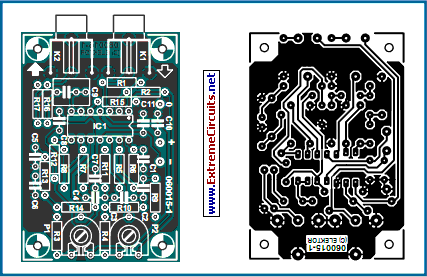# Learning Electronics

Learn to build electronic circuits

# Paraphase Tone Controller

As opposed to the widespread Baxandall circuit (dating back to 1952!) a ‘paraphrase’ tone control supplies a straight frequency response as long as the bass and treble controls are in the same position. This unique property makes the ‘paraphase’ configuration of interest if only treble or bass needs to be adjusted - it is not possible to adjust both at the same time! Essentially, it’s the difference in setting of the tone controls that determines the slope of the frequency response, and the degree of bass/treble correction. The circuit is simplicity itself, based on two networks C1-C2-C3/R9-R10-R11 and C5-C6-C7/R12-R13-R14.

Picture of the project:Paraphase Tone Controller Circuit

The first is for the high frequencies (treble) response, the second, for the low frequencies (bass). The roll-off points have been selected, in combination with C4 and C8, for the sum of the two output signals to re-appear with a ‘straight’ frequency response again at the output. Roughly equal output levels from the networks are ensured by R6 = 7.15 k and R8 = 6.80 k. However, the operating principle requires the input signals to the two networks to be in anti-phase. For best operation the networks are driven by two buffers providing some extra gain.

Circuit diagram:Paraphase Tone Controller Circuit Diagram

The gain of IC1.D is slightly higher than that of IC1.C to ensure the overall response curve remains as flat as possible at equal settings of the tone controls. Because each network introduces a loss of about 1.72 (times), IC1.D and IC1.C first amplify the signal. The gain is set at about 8 (times) allowing input signal levels up to 1 V to pass the circuit at maximum gain and distortion-free. The gain also compensates the attenuation if you prefer to keep the tone controls at the mid positions for a straight response.

Parts and PCB layout:Parts and PCB Layout

To audio fans, the circuit is rewarding to experiment with, especially in respect of the crossover point of the two networks. R3 and R4 determine the control range, which may be increased (within limits) by using lower resistor values here. The values shown ensure a tone control range of about 20 dB. IC1.B buffers the summed signal across R15. C9 removes any DC-offset voltage and R16 protects the output buffer from the effects of too high capacitive loads. R17, finally, keeps the output at 0 V.

The choice of the quad opamp is relatively uncritical. Here the unassuming TL074 is used but you may even apply rail to rail opamps as long as they are stable at unity gain. Also, watch the supply voltage range. A simple circuit board was designed for the project. Linear-law potentiometers may be fitted directly onto the board. Two boards are required for a stereo application. The relevant connections on the boards are then wired to a stereo control potentiometer.

Specification:
• Current consumption (no signal) 8 mA
• Max. input signal 1 Veff (at max. gain)
• Gain at 20 Hz +13.1 dB max. –6.9 dB min.
• at 20 kHz +12.2 dB max. –7.6 dB min
• Gain (controls at mid position) 2.38 x
• Distortion (1 Veff, 1 kHz) 0.002% (B = 22kHz) 0.005% (B = 80 kHz)
COMPONENTS LIST
Resistors
R1-R4 = 10k
R5,R7 = 1k
R6 = 7k15
R8 = 6k80
R9,R10,R11 = 8k2
R12,R13,R14 = 2k2
R15 = 1M
R16 = 100R
R17 = 100k
P1,P2 = 100k preset or chassis-
mount control potentiometer, linear law
Capacitors
C1,C2,C3 = 47nF MKT, lead pitch 5mm
C4 = 68nF MKT, lead pitch 5mm
C5,C6,C7 = 10nF MKT, lead pitch 5mm
C8,C10,C11 = 100nF MKT, lead pitch 5mm
C9 = 2µF2 MKT, lead pitch 5mm or 7.5mm
Semiconductors
IC1 = TL074
Miscellaneous
K1,K2 = line socket, PCB mount, e.g.
T-709G (Monacor/Monarch)
Author: Ton Giesberts - Copyright: Elektor Electronics